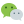# 命令式编程(Imperative)

``````function myFn(n) {
for (i = 0; i < 3; i++) {
n++;
}
console.log(n);
}
myFn(0);  // 3
``````

# 函数式编程/声明式(Functional/Declarative)

• 函数式编程是声明式的；
• 提倡纯函数理念，变量私有，不同于面向对象编程的成员共享；
• 无副作用，不影响其他外部变量；
• 有些类似初中数学纯函数f(x)的定义，提供输入值，返回新的输出值，每次提供相同输入值总能返回相同输出值，使线程安全可靠；
``````Array.map();    // 纯函数，输出唯一
Math.random();  // 非纯函数，输出不唯一
``````
• 函数的大量使用，变量中存储函数，动态创建函数，返回值为函数，函数作为参数传递，等等；
``````// 字符串通过函数储存在变量中
var myStr = function(){return 'Hello World'};
console.log(myStr);  // Hello World

// 对象属性值储存为函数
var myObj = {
name: 'Cloud',
getName: function(){
return this.name;
}
}
console.log(myObj.getName()); // Cloud

// 动态创建函数，用时调用，用完销毁
console.log('Hello ' + (function(){
return 'World';
})());  // Hello World

// 函数作为参数进行传递
function paraFn() {
return 'Hello';
}
function myFn(a, b) {
return a + b;
}
console.log(myFn(paraFn(), 'World')); // Hello World

// 函数作为返回值
function myFn2() {
var a = 'Hello ';
return function(){
return a + 'World';
}
}
console.log(myFn2()());  // Hello World
``````

``````// 命令式
var a = [1, 2, 3];
var b = [];
for (i = 0; i < 3; i++) {
b.push(a[i] * a[i]);
}
console.log(b);  // [1, 4, 9]

// 声明式
var a = [1, 2, 3];
var b = a.map(function(i){
return i * i;
});
console.log(b);  // [1, 4, 9]
``````

• 说明想要实现的功能，让机器完成步骤以及如何实现；

• 免去一些不必要的命令步骤，让思维集中在功能开发上，而不是冗长的复杂过程实现；

``````function factorial(n) {
if (n == 0) return 1;
return n * factorial(n-1);
}
console.log(factorial(3));  // 3 x 2 x 1 = 6
``````

.map() .reduce()等 也是申明式编程函数；

# 函数合成

``````// 高中数学常见的过程
g(x) = 2x;
h(x) = x + 3;
f(x) = 2x + 3;
// 则可变换为以下形式，即我们所学的复合函数
f(x) = h(g(x));
``````

js的实现：

``````function gFn(x) {
return x *2;
}
function hFn(x) {
return x + 3;
}
console.log(hFn(gFn(1)));  // 5

// 使用函数合成
function fFn(x) {
return hFn(gFn(x));
}
console.log(fFn(1));  // 5
``````

# 函数柯理化(Currying)

``````// 原函数
function plusFn(x, y, z) {
return x + y + z;
}
console.log(plusFn(1, 2, 3));  // 6

// 柯理化后
function plusFn(x) {
return function(y) {
return function(z) {
return x + y + z;
}
}
}
console.log(plusFn(1)(2)(3)); // 6
``````

### 评论：微信公众号：程序骑士